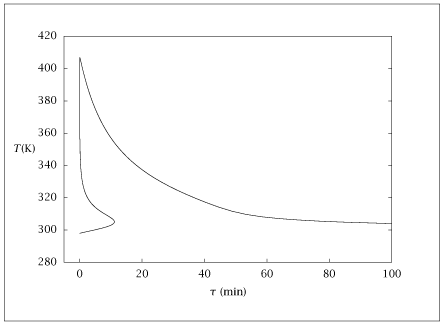## Figure 6.19:

### Steady-state temperature versus residence time.## Code for Figure 6.19

### main.m

```
1
2
3
4
5
6
7
8
9
10
11
12
13
14
15
16
17
18
19
20
21
22
23
24
25
26
27
28
29
30
31
32
33
34
35
36
37
38
39
40
41
42
43
44
45
46
47
48
49
50
51
52
53
54
55
56
57
58
59
60
61
62
63
64
65
66
67
68
69
70
71
72
73
74
75
76
77
78
79
80
81
82
83
84
85
86
87
88
89
90
91
92
93
94
95
96
97
98
99
100
101
102
103
104
105
106
107
108
109
110
111
112
113
114
115
116
117
118
119
120
121
122
123
124
125
126
127
128
129
130
131
132% Copyright (C) 2001, James B. Rawlings and John G. Ekerdt
%
% This program is free software; you can redistribute it and/or
% modify it under the terms of the GNU General Public License as
% published by the Free Software Foundation; either version 2, or (at
% your option) any later version.
%
% This program is distributed in the hope that it will be useful, but
% WITHOUT ANY WARRANTY; without even the implied warranty of
% MERCHANTABILITY or FITNESS FOR A PARTICULAR PURPOSE.  See the GNU
% General Public License for more details.
%
% You should have received a copy of the GNU General Public License
% along with this program; see the file COPYING.  If not, write to
% the Free Software Foundation, 59 Temple Place - Suite 330, Boston,
% MA 02111-1307, USA.

global k_m T_m E c_Af c_A theta C_ps T_f DeltaH_R U T_a
%
% limit cycle parameters
%
% units are kmol, min, kJ, K, m^3
%
% 9/10/98, jbr
%
k_m      = 0.004;
T_m      = 298;
E        = 15000;
c_Af     = 2;
C_p      = 4;
rho      = 1000;
C_ps     = C_p*rho;
T_f      = 298;
T_a      = T_f;
DeltaH_R = -2.2e5;
U        = 340;
theta    = 35;
T_set    = 321.53;
c_set    = 0.48995;
T_fs     = T_f;
Kc       = 0;
gamma    = E/T_f;
B        = -DeltaH_R*c_Af*gamma/(C_ps*T_f);
beta     = U/C_ps*theta;
Da       = k_m*exp(-E*(1/T_f-1/T_m))*theta;
x2c      = (T_a-T_f)/T_f*gamma;

%
% building the lower branch;
% use (theta,T) as dependent and
% c_A as independent
%
x0=[1; T_f];
nc_As = 200;
%tmp_table(1,:) = [0 T_f 0 -Inf -Inf 0];
c_Avect = linspace(0.9999*c_Af, .005*c_Af, nc_As);
for i = 1: nc_As
c_A = c_Avect(i);
opts = optimset ('MaxFunEvals', 2000*numel (x0), ...
'MaxIter', 500*numel (x0));
[x, fval, info] = fsolve('st_st_cA', x0, opts);
theta = x(1);
T     = x(2);
conv     = (c_Af - c_A) / c_Af;
%
% compute the eigenvalues of the Jacobian
%
k = k_m*exp(-E*(1/T - 1/T_m));
Jac = [-1/theta - k,     -k*c_A*E/(T*T);
-k*DeltaH_R/C_ps, -U/C_ps - 1/theta - k*c_A*DeltaH_R/C_ps*E/(T*T)];
% lambda = sort(real(eig(Jac)))';
lambda = eig(Jac);
lamrow = [real(lambda(1)) imag(lambda(1)) real(lambda(2)) ...
imag(lambda(2))];
tmp_table(i,:) = [theta, T, conv, lamrow, info];
x0=x;
end
table = [tmp_table];

%
% turning the corner on the upper branch;
% switch to (c_A,T) as dependent and
% theta as independent
% load x with current solution
%
x0=[c_A; T];
cortheta= theta;
nthetas = 100;
theta_vect = logspace(log10(cortheta), log10(500), nthetas);
clear tmp_table;
for i = 1: nthetas
theta = theta_vect(i);
opts = optimset ('MaxFunEvals', 2000*numel (x0), ...
'MaxIter', 500*numel (x0));
[x, fval, info] = fsolve('st_st_theta', x0, opts);
c_A   = x(1);
T     = x(2);
conv     = (c_Af - c_A) / c_Af;
k = k_m*exp(-E*(1/T - 1/T_m));
%
% compute the eigenvalues of the Jacobian
%
Jac = [-1/theta - k,     -k*c_A*E/(T*T);
-k*DeltaH_R/C_ps, -U/C_ps - 1/theta - k*c_A*DeltaH_R/C_ps*E/(T*T)];
% lambda = sort(real(eig(Jac)))';
lambda = eig(Jac);
lamrow = [real(lambda(1)) imag(lambda(1)) real(lambda(2)) ...
imag(lambda(2))];
tmp_table(i,:) = [theta, T, conv, lamrow, info];
x0=x;
end

table = [table; tmp_table];
save -ascii st_st_osc.dat table;

if (~ strcmp (getenv ('OMIT_PLOTS'), 'true')) % PLOTTING
plot(table(:,1),table(:,3));
axis ([-5,100,0,1]);

figure()
semilogx(table(:,1),table(:,3));
axis([.001,1000,0,1]);

figure()
plot(table(:,1),table(:,2));
axis ([-5,100,280,420]);

figure()
semilogx(table(:,1),table(:,2));
axis([.001,1000,280,420]);
% TITLE
end % PLOTTING

```

### st_st_cA.m

```
1
2
3
4
5
6
7function retval = st_st_cA(x)
global k_m T_m E c_Af c_A theta C_ps T_f DeltaH_R U T_a
theta = x(1);
T     = x(2);
k         = k_m*exp(-E*(1/T - 1/T_m));
retval(1) = c_Af - (1+k*theta)*c_A;
retval(2) = U*theta*(T_a - T) + C_ps*(T_f - T) - k*theta*c_A*DeltaH_R;

```

### st_st_theta.m

```
1
2
3
4
5
6
7function retval = st_st_theta(x)
global k_m T_m E c_Af c_A theta C_ps T_f DeltaH_R U T_a
c_A   = x(1);
T     = x(2);
k         = k_m*exp(-E*(1/T - 1/T_m));
retval(1) = c_Af - (1+k*theta)*c_A;
retval(2) = U*theta*(T_a - T) + C_ps*(T_f - T) - k*theta*c_A*DeltaH_R;

```